Next: Rotational Flattening Up: Gravitational Potential Theory Previous: Potential Due to a

Potential Outside a Uniform Spheroid

Let us now calculate the gravitational potential generated outside a spheroid of uniform mass density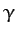and mean radius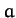. A spheroid is the solid body produced by rotating an ellipse about a major or minor axis. Let the axis of rotation coincide with the-axis, and let the outer boundary of the spheroid satisfy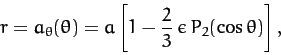(901)

whereis the termed the ellipticity. Here, we are assuming that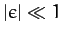, so that the spheroid is very close to being a sphere. If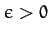then the spheroid is slightly squashed along its symmetry axis, and is termed oblate. Likewise, if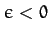then the spheroid is slightly elongated along its axis, and is termed prolate--see Figure 39. Of course, if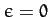then the spheroid reduces to a sphere.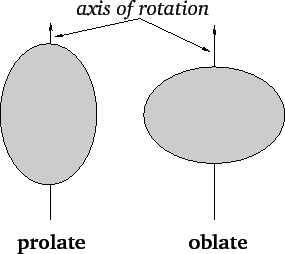Now, according to Equation (889) and (890), the gravitational potential generated outside an axially symmetric mass distribution can be written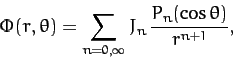(902)

where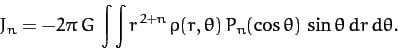(903)

Here, the integral is taken over the whole cross-section of the distribution in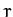-space.

It follows that for a uniform spheroid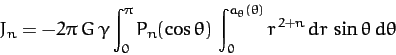(904)

Hence,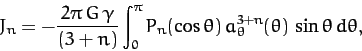(905)

giving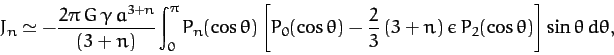(906)

to first-order in. It is thus clear, from Equation (886), that, to first-order in, the only non-zero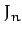are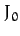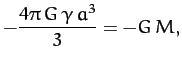(907)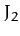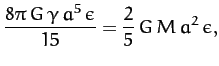(908)

since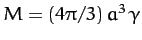.

Thus, the gravitational potential outside a uniform spheroid of total mass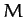, mean radius, and ellipticity, is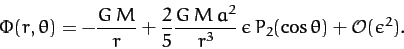(909)

In particular, the gravitational potential on the surface of the spheroid is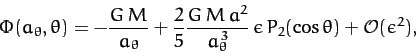(910)

which yields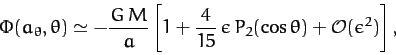(911)

where use has been made of Equation (901).

Consider a self-gravitating spheroid of mass, mean radius, and ellipticity: e.g., a star, or a planet. Assuming, for the sake of simplicity, that the spheroid is composed of uniform density incompressible fluid, the gravitational potential on its surface is given by Equation (911). However, the condition for an equilibrium state is that the potential be constant over the surface. If this is not the case then there will be gravitational forces acting tangential to the surface. Such forces cannot be balanced by internal pressure, which only acts normal to the surface. Hence, from (911), it is clear that the condition for equilibrium is. In other words, the equilibrium configuration of a self-gravitating mass is a sphere. Deviations from this configuration can only be caused by forces in addition to self-gravity and internal pressure: e.g., centrifugal forces due to rotation, or tidal forces due to orbiting masses.Next: Rotational Flattening Up: Gravitational Potential Theory Previous: Potential Due to a
Richard Fitzpatrick 2011-03-31